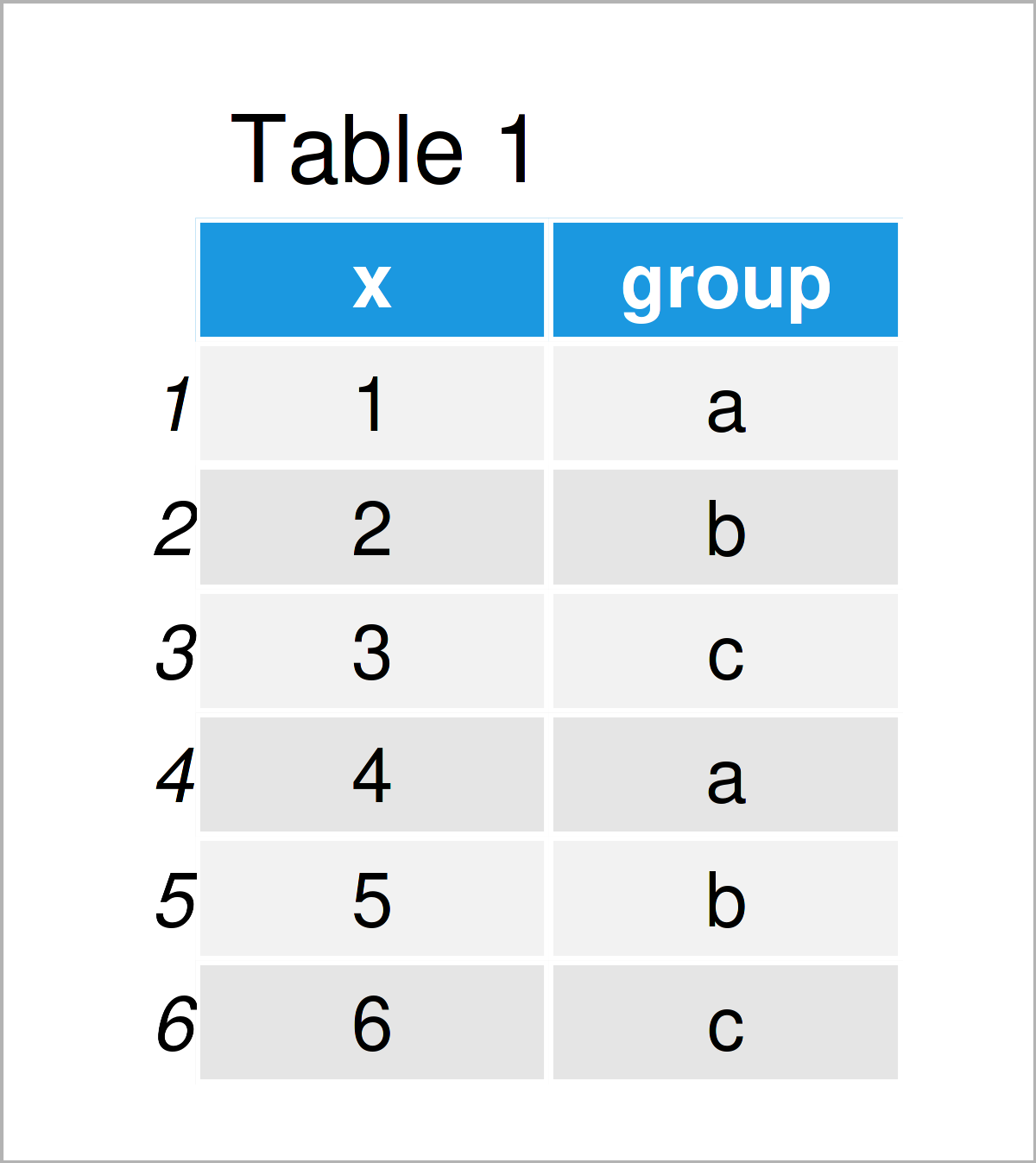# Error in unique.default(x, nmax = nmax) : unique() applies only to vectors in R (2 Examples)

In this article you’ll learn how to handle the “Error in unique.default(x, nmax = nmax) : unique() applies only to vectors” in R.

You’re here for the answer, so let’s get straight to the examples:

## Exemplifying Data

Let’s first create some example data:

```data <- data.frame(x = 1:6,            # Create example data
group = letters[1:3])
data                                   # Print example data```As you can see based on Table 1, the example data is a data frame having six rows and two columns. The variable x has the integer class and the variable group has the character class.

## Example 1: Reproduce the Error in unique.default(x, nmax = nmax) : unique() applies only to vectors

In this section, I’ll show how to replicate the error message “Error in unique.default(x, nmax = nmax) : unique() applies only to vectors”.

Let’s assume that we want to calculate group averages using the ave function. Then, we might try to run the R code below:

```ave(data\$x, data\$group, mean)          # Code leads to error
# Error in unique.default(x, nmax = nmax) :
#   unique() applies only to vectors```

Unfortunately, the error message “Error in unique.default(x, nmax = nmax) : unique() applies only to vectors” was returned.

This is because we have not specified the FUN argument explicitly. Let’s fix this!

## Example 2: Debug the Error in unique.default(x, nmax = nmax) : unique() applies only to vectors

The following R programming syntax illustrates how to avoid the “Error in unique.default(x, nmax = nmax) : unique() applies only to vectors” when using the ave function.

For this, we have to specify the mean function to the named argument FUN:

```ave(data\$x, data\$group, FUN = mean)    # Code works fine
#  2.5 3.5 4.5 2.5 3.5 4.5```

This time, it worked flawlessly!

## Video & Further Resources

Do you want to know more about the handling of the “Error in unique.default(x, nmax = nmax) : unique() applies only to vectors”? Then you may have a look at the following video on my YouTube channel. In the video instruction, I’m explaining the contents of this post in RStudio.

In addition, you might have a look at the other articles on my homepage:

In this article, I have shown how to reproduce and fix the “Error in unique.default(x, nmax = nmax) : unique() applies only to vectors” in the R programming language. In case you have any additional questions, don’t hesitate to let me know in the comments section. Furthermore, don’t forget to subscribe to my email newsletter to receive regular updates on new tutorials.

Subscribe to the Statistics Globe Newsletter